## Why perform strain analysis?

Why do we perform strain analysis?. It can be important to retrieve information about strain from deformed rocks. First of all, strain analysis gives us an opportunity to explore the state of strain in a rock and to map out strain variations in a sample, an outcrop or a region. Strain data are important in the mapping and understanding of shear zones in orogenic belts. Strain measurements can also be used to estimate the amount of offset across a shear zone. It is possible to extract important information from shear zones if strain is known.
In many cases it is useful to know if the strain is planar or three dimensional. If planar, an important criterion for section balancing is fulﬁlled, be it across orogenic zones or extensional basins. The shape of the strain ellipsoid may also contain information about how the deformation occurred. Oblate (pancake-shaped) strain in an orogenic setting may, for example, indicate ﬂattening strain related to gravity-driven collapse rather than classic push-from-behind thrusting.
The orientation of the strain ellipsoid is also important, particularly in relation to rock structures. In a shear zone setting, it may tell us if the deformation was simple shear or not. Strain in folded layers helps us to understand fold-forming mechanism(s). Studies of deformed reduction spots in slates give good estimates on how much shortening has occurred across the foliation in such rocks, and strain markers in sedimentary rocks can sometimes allow for reconstruction of original sedimentary thickness.

### Strain in one dimension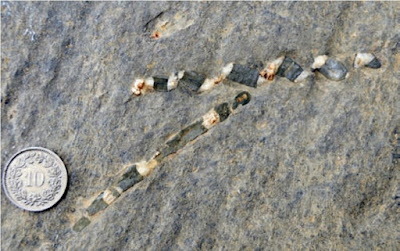Two elongated belemnites in Jurassic limestone in the Swiss Alps. The different ways that the two belemnites have been stretched give us some two-dimensional information about the strain ﬁeld: the upper belemnite has experienced sinistral shear strain while the lower one has not and must be close to the maximum stretching direction.
One-dimensional strain analyses are concerned with changes in length and therefore the simplest form of strain analysis we have. If we can reconstruct the original length of an object or linear structure we can also calculate the amount of stretching or shortening in that direction. Objects revealing the state of strain in a deformed rock are known as strain markers. Examples of strain markers indicating change in length are boudinaged dikes or layers, and minerals or linear fossils such as belemnites or graptolites that have been elongated, such as the stretched Swiss belemnites shown in Figure above. Or it could be a layer shortened by folding. It could even be a faulted reference horizon on a geologic or seismic proﬁle. The horizon may be stretched by normal faults or shortened by reverse faults, and the overall strain is referred to as brittle strain. One-dimensional strain is revealed when the horizon, fossil, mineral or dike is restored to its pre-deformational state.

### Strain in two dimensions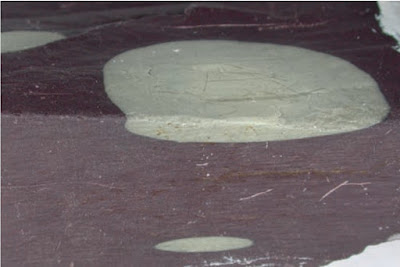Reduction spots in Welsh slate. The light spots formed as spherical volumes of bleached (chemically reduced) rock. Their new shapes are elliptical in cross-section and oblate (pancake-shaped) in three dimensions, reﬂecting the tectonic strain in these slates.
In two-dimensional strain analyses we look for sections that have objects of known initial shape or contain linear markers with a variety of orientations (Figure first). Strained reduction spots of the type shown in Figure above are perfect, because they tend to have spherical shapes where they are undeformed. There are also many other types of objects that can be used, such as sections through conglomerates, breccias, corals, reduction spots, oolites, vesicles, pillow lavas (Figure below), columnar basalt, plutons and so on. Two-dimensional strain can also be calculated from one-dimensional data that represent different directions in the same section. A typical example would be dikes with different orientations that show different amounts of extension.Section through a deformed Ordovician pahoe-hoe lava. The elliptical shapes were originally more circular, and Hans Reusch, who made the sketch in the 1880s, understood that they had been ﬂattened during deformation. The Rf/f, center-to-center, and Fry methods would all be worth trying in this case.
Strain extracted from sections is the most common type of strain data, and sectional data can be combine to estimate the three-dimensional strain ellipsoid.

#### Changes in angles

Strain can be found if we know the original angle between sets of lines. The original angular relations between structures such as dikes, foliations and bedding are sometimes found in both undeformed and deformed states, i.e. outside and inside a deformation zone. We can then see how the strain has affected the angular relationships and use this information to estimate strain. In other cases orthogonal lines of symmetry found in undeformed fossils such as trilobites, brachiopods and worm burrows (angle with layering) can be used to determine the angular shear in some deformed sedimentary rocks. In general, all we need to know is the change in angle between sets of lines and that there is no strain partitioning due to contrasting mechanical properties of the objects with respect to the enclosing rock.

If the angle was 90 degree in the undeformed state, the change in angle is the local angular shear. The two originally orthogonal lines remain orthogonal after the deformation, then they must represent the principal strains and thus the orientation of the strain ellipsoid. Observations of variously oriented line sets thus give information about the strain ellipse or ellipsoid. All we need is a useful method. Two of the most common methods used to ﬁnd strain from initially orthogonal lines are known as the Wellman and Breddin methods, and are presented in the following sections.

#### The Wellman methodWellman’s method involves construction of the strain ellipse by drawing parallelograms based on the orientation of originally orthogonal pairs of lines. The deformation was produced on a computer and is a homogeneous simple shear. However, the strain ellipse itself tells us nothing about the degree of coaxiality: the same result could have been attained by pure shear.
This method dates back to 1962 and is a geometric construction for ﬁnding strain in two dimensions (in a section). It is typically demonstrated on fossils with orthogonal lines of symmetry in the undeformed state. In Figure above a we use the hinge and symmetry lines of brachiopods. A line of reference must be drawn (with arbitrary orientation) and pairs of lines that were orthogonal in the unstrained state are identiﬁed. The reference line must have two deﬁned endpoints, named A and B in Figure above b. A pair of lines is then drawn parallel to both the hinge line and symmetry line for each fossil, so that they intersect at the endpoints of the reference line. The other points of intersection are marked (numbered 1–6 in Figure above b, c). If the rock is unstrained, the lines will deﬁne rectangles. If there is a strain involved, they will deﬁne parallelograms. To ﬁnd the strain ellipse, simply ﬁt an ellipse to the numbered corners of the parallelograms (Figure above c). If no ellipse can be ﬁtted to the corner points of the rectangles the strain is heterogeneous or, alternatively, the measurement or assumption of initial orthogonality is false. The challenge with this method is, of course, to ﬁnd enough fossils or other features with initially orthogonal lines typically 6–10 are needed.

#### The Breddin graphThe data from the previous ﬁgure plotted in a Breddin graph. The data points are close to the curve for R=2.5.
We have already stated that the angular shear depends on the orientation of the principal strains: the closer the deformed orthogonal lines are to the principal strains, the lower the angular shear. This fact is utilized in a method ﬁrst published by Hans Breddin in 1956 in German (with some errors). It is based on the graph shown in Figure above, where the angular shear changes with orientation and strain magnitude R. Input are the angular shears and the orientations of the sheared line pairs with respect to the principal strains. These data are plotted in the so-called Breddin graph and the R-value (ellipticity of the strain ellipse) is found by inspection (Figure above). This method may work even for only one or two observations.
In many cases the orientation of the principal axes is unknown. In such cases the data are plotted with respect to an arbitrarily drawn reference line. The data are then moved horizontally on the graph until they ﬁt one of the curves, and the orientations of the strain axes are then found at the intersections with the horizontal axis (Figure above). In this case a larger number of data are needed for good results.

#### Elliptical objects and the Rf/f-method

Objects with initial circular (in sections) or spherical (in three dimensions) geometry are relatively uncommon, but do occur. Reduction spots and ooliths perhaps form the most perfect spherical shapes in sedimentary rocks. When deformed homogeneously, they are transformed into ellipses and ellipsoids that reﬂect the local ﬁnite strain. Conglomerates are perhaps more common and contain clasts that reﬂect the ﬁnite strain. In contrast to oolites and reduction spots, few pebbles or cobbles in a conglomerate are spherical in the undeformed state. This will of course inﬂuence their shape in the deformed state and causes a challenge in strain analyses. However, the clasts tend to have their long axes in a spectrum of orientations in the undeformed state, in which case methods such as the Rf/f-method may be able to take the initial shape factor into account.The Rf/f method illustrated. The ellipses have the same ellipticity (Ri) before the deformation starts. The Rf–f diagram to the right indicates that Ri=2. A pure shear is then added with Rs=1.5 followed by a pure shear strain of Rs=3. The deformation matrices for these two deformations are shown. Note the change in the distribution of points in the diagrams to the right. Rs in the diagrams is the actual strain that is added. Modiﬁed from Ramsay and Huber (1983).
The Rf/f-method was ﬁrst introduced by John Ramsay in his well-known 1967 textbook and was later improved. The method is illustrated in Figure above. The markers are assumed to have approximately elliptical shapes in the deformed (and undeformed) state, and they must show a signiﬁcant variation in orientations for the method to work.
The Rf/f-method handles initially non-spherical markers, but the method requires a signiﬁcant variation in the orientations of their long axes.
The ellipticity (X/Y) in the undeformed (initial) state is called Ri. In our example (Figure above) Ri=2. After a strain Rs the markers exhibit new shapes. The new shapes are different and depend on the initial orientation of the elliptical markers. The new (ﬁnal) ellipticity for each deformation marker is called Rf and the spectrum of Rf-values is plotted against their orientations, or more speciﬁcally against the angle f' between the long axis of the ellipse and a reference line (horizontal in Figure above). In our example we have applied two increments of pure shear to a series of ellipses with different orientations. All the ellipses have the same initial shape Ri=2, and they plot along a vertical line in the upper right diagram in Figure above. Ellipse 1 is oriented with its long axis along the minimum principal strain axis, and it is converted into an ellipse that shows less strain (lower Rf-value) than the true strain ellipse (Rs). Ellipse 7, on the other hand, is oriented with its long axis parallel to the long axis of the strain ellipse, and the two ellipticities are added. This leads to an ellipticity that is higher than Rs. When Rs=3, the true strain Rs is located somewhere between the shape represented by ellipses 1 and 7, as seen in Figure above (lower right diagram).
For Rs=1.5 we still have ellipses with the full spectrum of orientations ( 90 to 90 ; see middle diagram in Figure above), while for Rs=3 there is a much more limited spectrum of orientations (lower graph in Figure above). The scatter in orientation is called the ﬂuctuation F. An important change happens when ellipse 1, which has its long axis along the Z-axis of the strain ellipsoid, passes the shape of a circle (Rs=Ri,) and starts to develop an ellipse whose long axis is parallel to X. This happens when Rs=2, and for larger strains the data points deﬁne a circular shape. Inside this shape is the strain Rs that we are interested in. But where exactly is Rs? A simple average of the maximum and minimum Rf-values would depend on the original distribution of orientations. Even if the initial distribution is random, the average R-value would be too high, as high values tend to be over represented (Figure above, lower graph).
To ﬁnd Rs we have to treat the cases where Rs >Ri and Rs <Ri separately. In the latter case, which is represented by the middle graph in Figure above, we have the following expressions for the maximum and minimum value for Rf:
In both cases the orientation of the long (X) axis of the strain ellipse is given by the location of the maximum Rf-values. Strain could also be found by ﬁtting the data to pre-calculated curves for various values for Ri and Rs. In practice, such operations are most efﬁciently done by means of computer programs.
The example shown in Figure above and discussed above is idealized in the sense that all the undeformed elliptical markers have identical ellipticity. What if this were not the case, i.e. some markers were more elliptical than others? Then the data would not have deﬁned a nice curve but a cloud of points in the Rf/f-diagram. Maximum and minimum Rf-values could still be found and strain could be calculated using the equations above. The only change in the equation is that Ri now represents the maximum ellipticity present in the undeformed state.
Another complication that may arise is that the initial markers may have had a restricted range of orientations. Ideally, the Rf/f-method requires the elliptical objects to be more or less randomly oriented prior to deformation. Conglomerates, to which this method commonly is applied, tend to have clasts with a preferred orientation. This may result in an Rf–f plot in which only a part of the curve or cloud is represented. In this case the maximum and minimum Rf-values may not be representative, and the formulas above may not give the correct answer and must be replaced by a computer based iterative retrodeformation method where X is input. However, many conglomerates have a few clasts with initially anomalous orientations that allow the use of Rf/f analysis.

#### Center-to-center method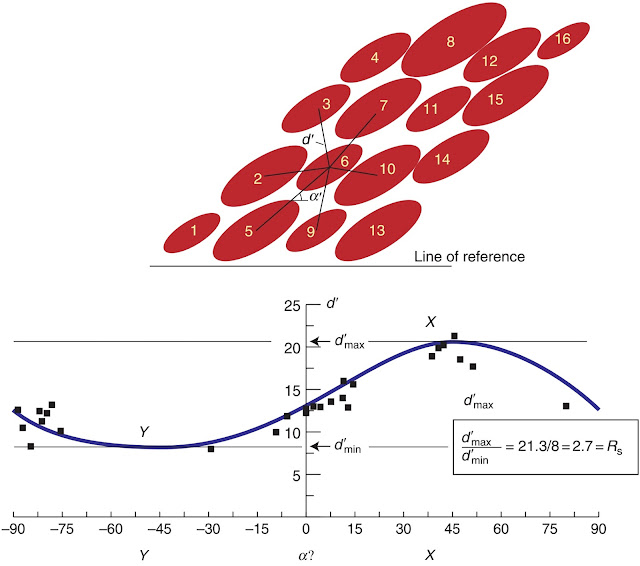The center-to-center method. Straight lines are drawn between neighbouring object centers. The length of each line (d') and the angle (a') that they make with a reference line are plotted in the diagram. The data deﬁne a curve that has a maximum at X and a minimum at the Y-value of the strain ellipse, and where Rs= X/Y.
This method, here demonstrated in Figure above, is based on the assumption that circular objects have a more or less statistically uniform distribution in our section(s). This means that the distances between neighboring particle centers were fairly constant before deformation. The particles could represent sand grains in well-sorted sandstone, pebbles, ooids, mud crack centers, pillow-lava or pahoe-hoe lava centers, pluton centers or other objects that are of similar size and where the centers are easily deﬁnable. If you are uncertain about how closely your section complies with this criterion, try anyway. If the method yields a reasonably well-deﬁned ellipse, then the method works.
The method itself is simple and is illustrated in Figure above: Measure the distance and direction from the center of an ellipse to those of its neighbours. Repeat this for all ellipses and graph the distance d' between the centers and the angles a' between the center tie lines and a reference line. A straight line occurs if the section is unstrained, while a deformed section yields a curve with maximum (d' max) and minimum values (d' min). The ellipticity of the strain ellipse is then given by the ratio: Rs =( d' max)/(d' min).

#### The Fry methodThe Fry method performed manually. (a) The centerpoints for the deformed objects are transferred to a transparent overlay. A central point (1 on the ﬁgure) is deﬁned. (b) The transparent paper is then moved to another of the points (point 2) and the centerpoints are again transferred onto the paper (the overlay must not be rotated). The procedure is repeated for all of the points, and the result (c) is an image of the strain ellipsoid (shape and orientation). Based on Ramsay and Huber (1983).
A quicker and visually more attractive method for ﬁnding two-dimensional strain was developed by Norman Fry at the end of the 1970s. This method, illustrated in Figure above, is based on the center-to-center method and is most easily dealt with using one of several available computer programs. It can be done manually by placing a tracing overlay with a coordinate origin and pair of reference axes on top of a sketch or picture of the section. The origin is placed on a particle center and the centers of all other particles (not just the neighbours) are marked on the tracing paper. The tracing paper is then moved, without rotating the paper with respect to the section, so that the origin covers a second particle center, and the centers of all other particles are again marked on the tracing paper. This procedure is repeated until the area of interest has been covered. For objects with a more or less uniform distribution the result will be a visual representation of the strain ellipse.The ellipse is the void area in the middle, deﬁned by the point cloud around it (Figure above c).
The Fry method, as well as the other methods presented in this section, outputs two-dimensional strain. Three-dimensional strain is found by combining strain estimates from two or more sections through the deformed rock volume. If sections can be found that each contain two of the principal strain axes, then two sections are sufﬁcient. In other cases three or more sections are needed, and the three-dimensional strain must be calculated by use of a computer.

### Strain in three dimensionsThree-dimensional strain expressed as ellipses on different sections through a conglomerate. The foliation (XY-plane) and the lineation (X-axis) are annotated. This illustration was published in 1888, but what are now routine strain methods were not developed until the 1960s.
A complete strain analysis is three-dimensional. Three dimensional strain data are presented in the Flinn diagram or similar diagrams that describe the shape of the strain ellipsoid, also known as the strain geometry. In addition, the orientation of the principal strains can be presented by means of stereographic nets. Direct ﬁeld observations of three-dimensional strain are rare. In almost all cases, analysis is based on two-dimensional strain observations from two or more sections at the same locality (Figure above). A well-known example of three-dimensional strain analysis from deformed conglomerates is presented in below heading.
In order to quantify ductile strain, be it in two or three dimensions, the following conditions need to be met:
The strain must be homogeneous at the scale of observation, the mechanical properties of the objects must have been similar to those of their host rock during the deformation, and we must have a reasonably good knowledge about the original shape of strain markers.
The strain must be homogeneous at the scale of observation, the mechanical properties of the objects must have been similar to those of their host rock during the deformation, and we must have a reasonably good knowledge about the original shape of strain markers.
The second point is an important one. For ductile rocks it means that the object and its surroundings must have had the same competence or viscosity. Otherwise the strain recorded by the object would be different from that of its surroundings. This effect is one of several types of strain partitioning, where the overall strain is distributed unevenly in terms of intensity and/or geometry in a rock volume. As an example, we mark a perfect circle on a piece of clay before ﬂattening it between two walls. The circle transforms passively into an ellipse that reveals the two-dimensional strain if the deformation is homogeneous. If we embed a coloured sphere of the same clay, then it would again deform along with the rest of the clay, revealing the three-dimensional strain involved. However, if we put a stiff marble in the clay the result is quite different. The marble remains unstrained while the clay around it becomes more intensely and heterogeneously strained than in the previous case. In fact, it causes a more heterogeneous strain pattern to appear. Strain markers with the same mechanical properties as the surroundings are called passive strain markers because they deform passively along with their surroundings. Those that have anomalous mechanical properties respond differently than the surrounding medium to the overall deformation, and such markers are called active strain markers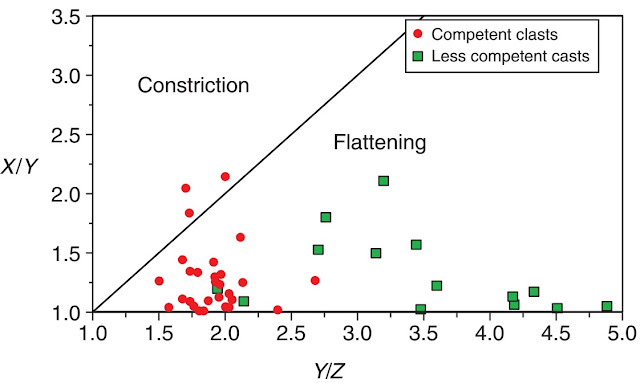Strain obtained from deformed conglomerates, plotted in the Flinn diagram. Different pebble types show different shapes and ﬁnite strains. Polymict conglomerate of the Utslettefjell Formation, Stord, southwest Norway.
An example of data from active strain markers is shown in Figure above. These data were collected from a deformed polymictic conglomerate where three-dimensional strain has been estimated from different clast types in the same rock and at the same locality. Clearly, the different clast types have recorded different amounts of strain. Competent (stiff) granitic clasts are less strained than less competent greenstone clasts. This is seen using the fact that strain intensity generally increases with increasing distance from the origin in Flinn space. But there is another interesting thing to note from this ﬁgure: It seems that competent clasts plot higher in the Flinn diagram (Figure. above) than incompetent(“soft”) clasts, meaning that competent clasts take on a more prolate shape. Hence, not only strain intensity but also strain geometry may vary according to the mechanical properties of strain markers.
The way that the different markers behave depends on factors such as their mineralogy, preexisting fabric, grain size, water content and temperature-pressure conditions at the time of deformation. In the case of Figure above, the temperature-pressure regime is that of lower to middle greenschist facies. At higher temperatures, quartz-rich rocks are more likely to behave as “soft” objects, and the relative positions of clast types in Flinn space are expected to change.
The last point above also requires attention: the initial shape of a deformed object clearly inﬂuences its postdeformational shape. If we consider two-dimensional objects such as sections through oolitic rocks, sandstones or conglomerates, the Rf/f method discussed above can handle this type of uncertainty. It is better to measure up two or more sections through a deformed rock using this method than dig out an object and measure its three-dimensional shape. The single object could have an unexpected initial shape (conglomerate clasts are seldom perfectly spherical or elliptical), but by combining numerous measurements in several sections we get a statistical variation that can solve or reduce this problem.
Three-dimensional strain is usually found by combining two-dimensional data from several differently oriented sections.
There are now computer programs that can be used to extract three-dimensional strain from sectional data. If the sections each contain two of the principal strain axes everything becomes easy, and only two are strictly needed (although three would still be good). Otherwise, strain data from at least three sections are required.

### Deformed quartzite conglomerates

Quartz or quartzite conglomerates with a quartzite matrix are commonly used for strain analyses. The more similar the mineralogy and grain size of the matrix and the pebbles, the less deformation partitioning and the better the strain estimates. A classic study of deformed quartzite conglomerates is Jake Hossack’s study of the Norwegian Bygdin conglomerate, published in 1968. Hossack was fortunate he found natural sections along the principal planes of the strain ellipsoid at each locality. Putting the sectional data together gave the three dimensional state of strain (strain ellipsoid) for each locality. Hossack found that strain geometry and intensity varies within his ﬁeld area. He related the strain pattern to static ﬂattening under the weight of the overlying Caledonian Jotun Nappe. Although details of his interpretation may be challenged, his work demonstrates how conglomerates can reveal a complicated strain pattern that otherwise would have been impossible to map.
Hossack noted the following sources of error:
• Inaccuracy connected with data collection (sections not being perfectly parallel to the principal planes of strain and measuring errors).
• Variations in pebble composition.
• The pre-deformational shape and orientation of the pebbles.
• Viscosity contrasts between clasts and matrix.
• Volume changes related to the deformation (pressure solution).
• The possibility of multiple deformation events.
Credits: Haakon Fossen (Structural Geology)

1.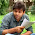2.